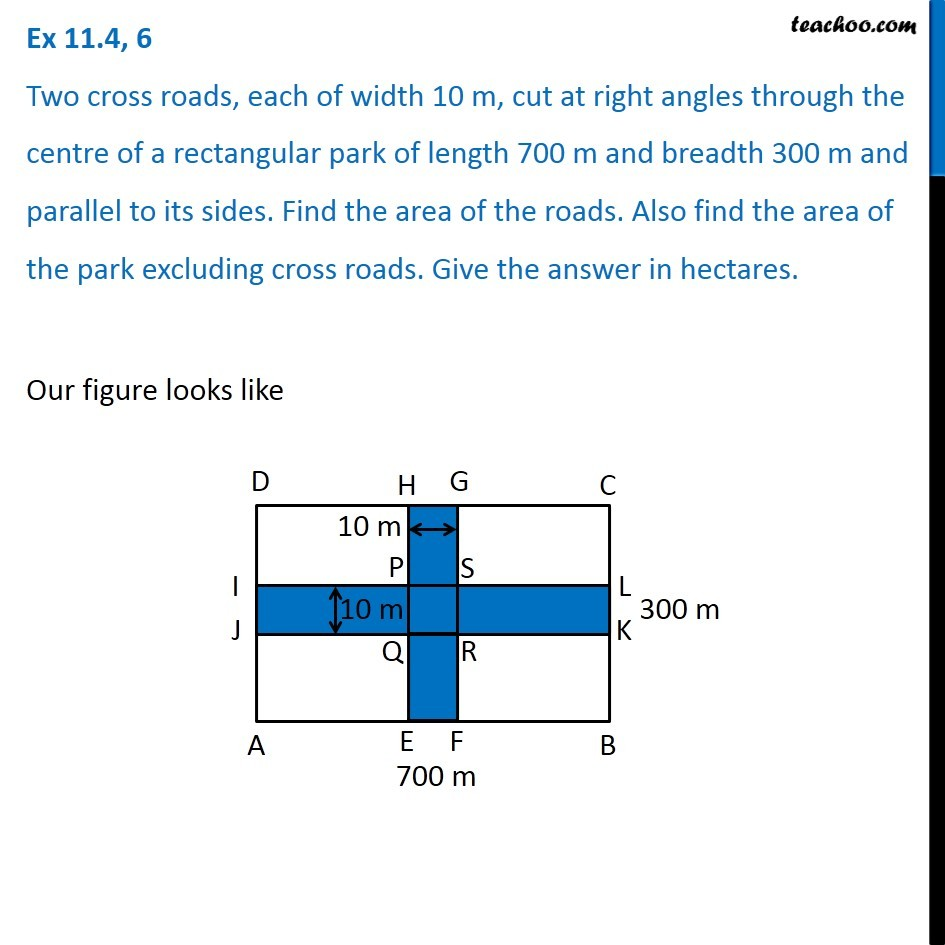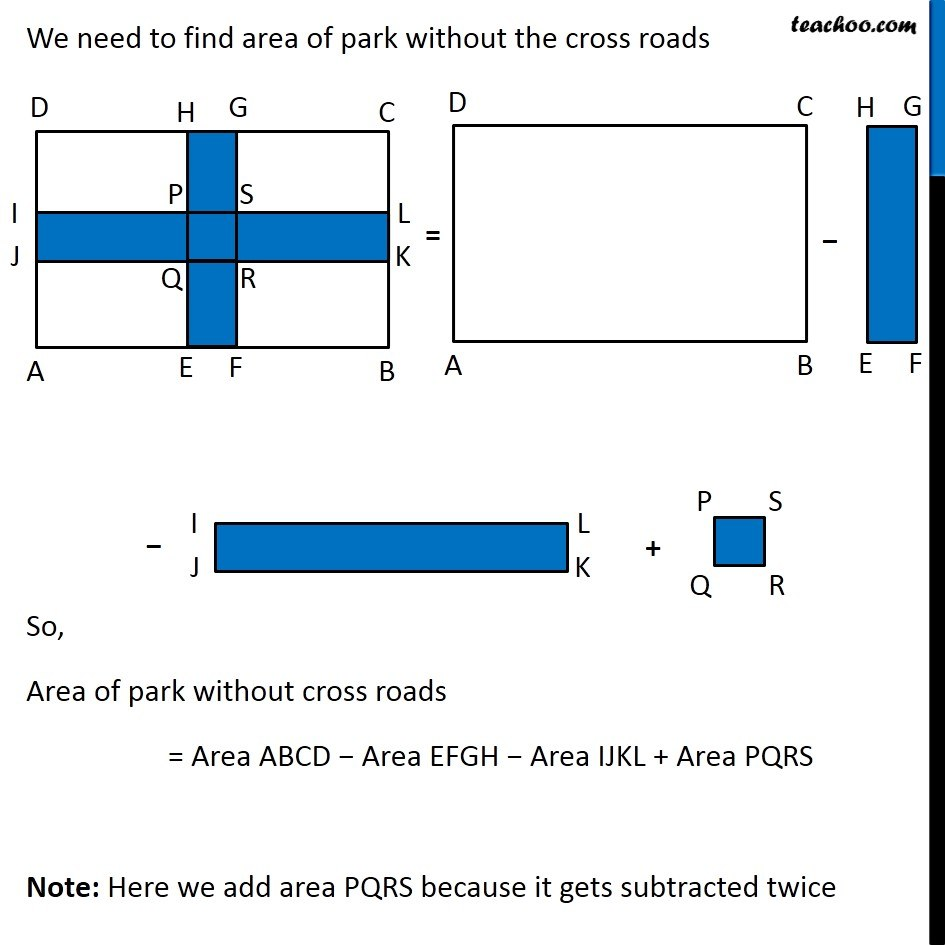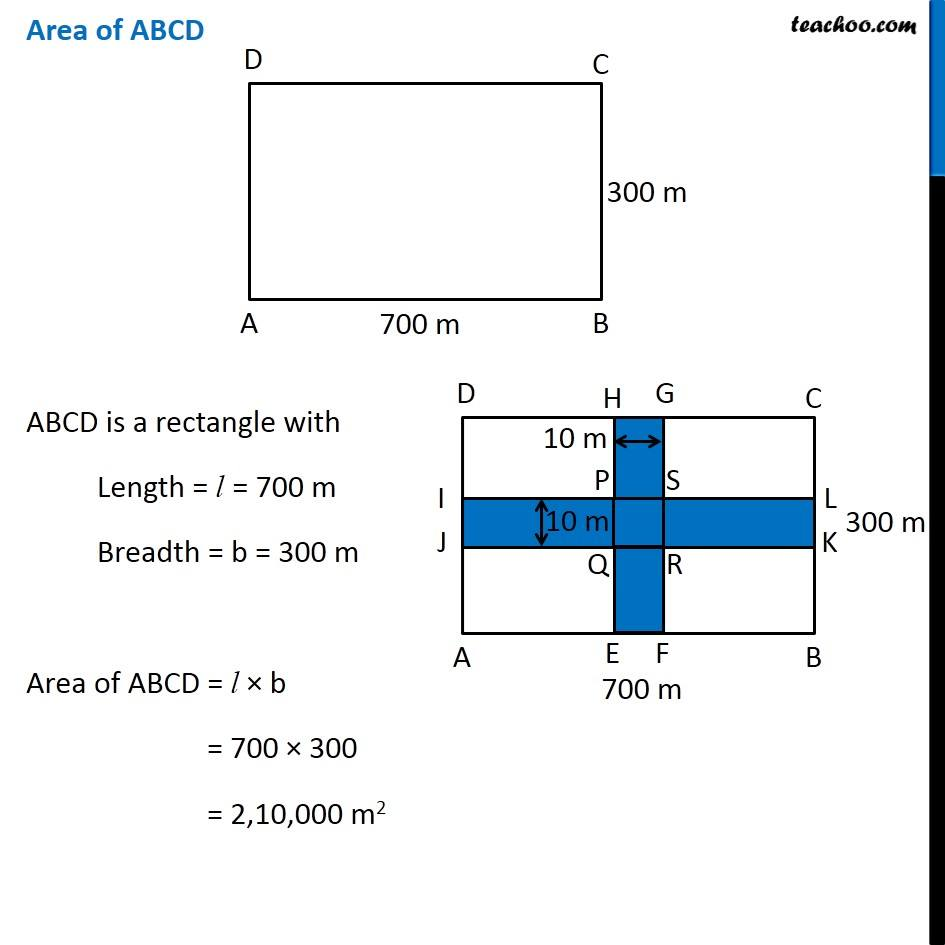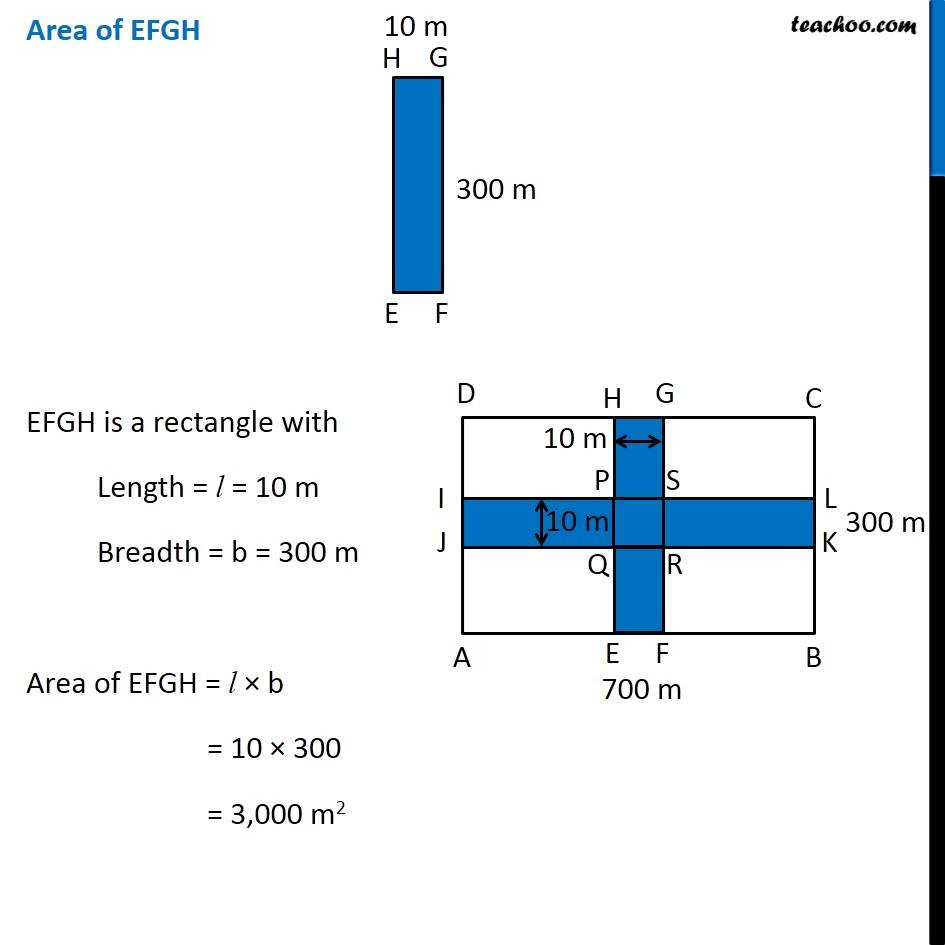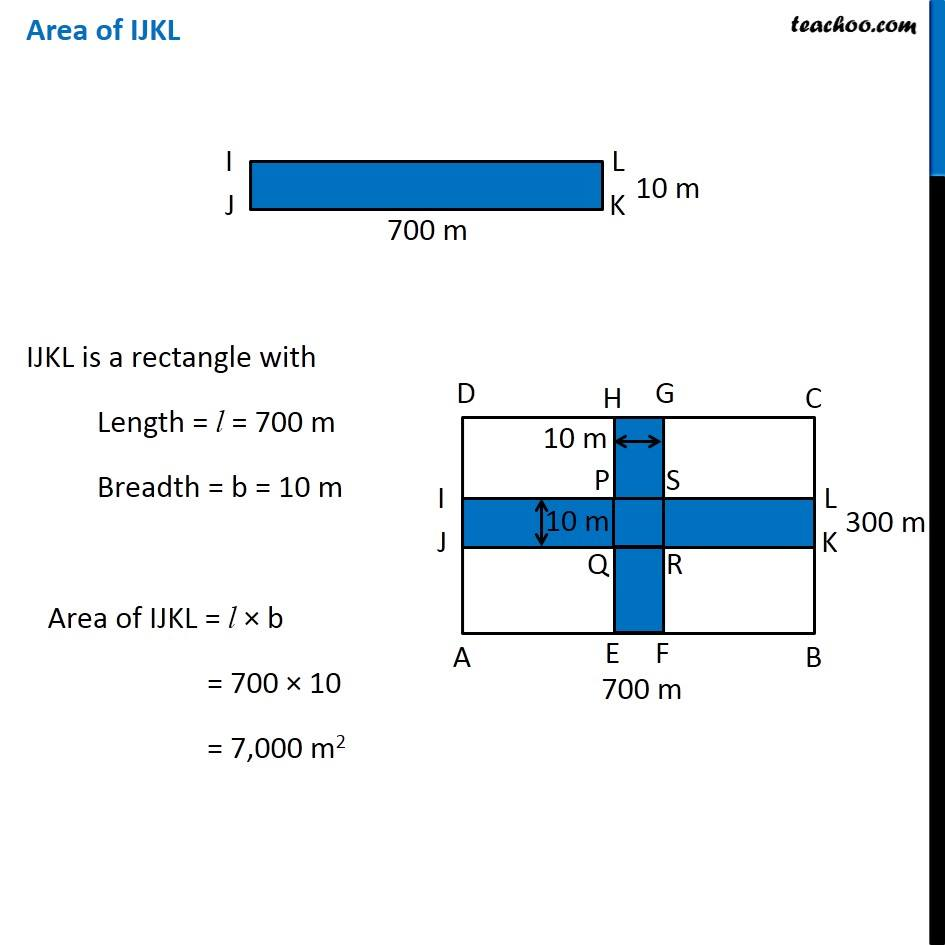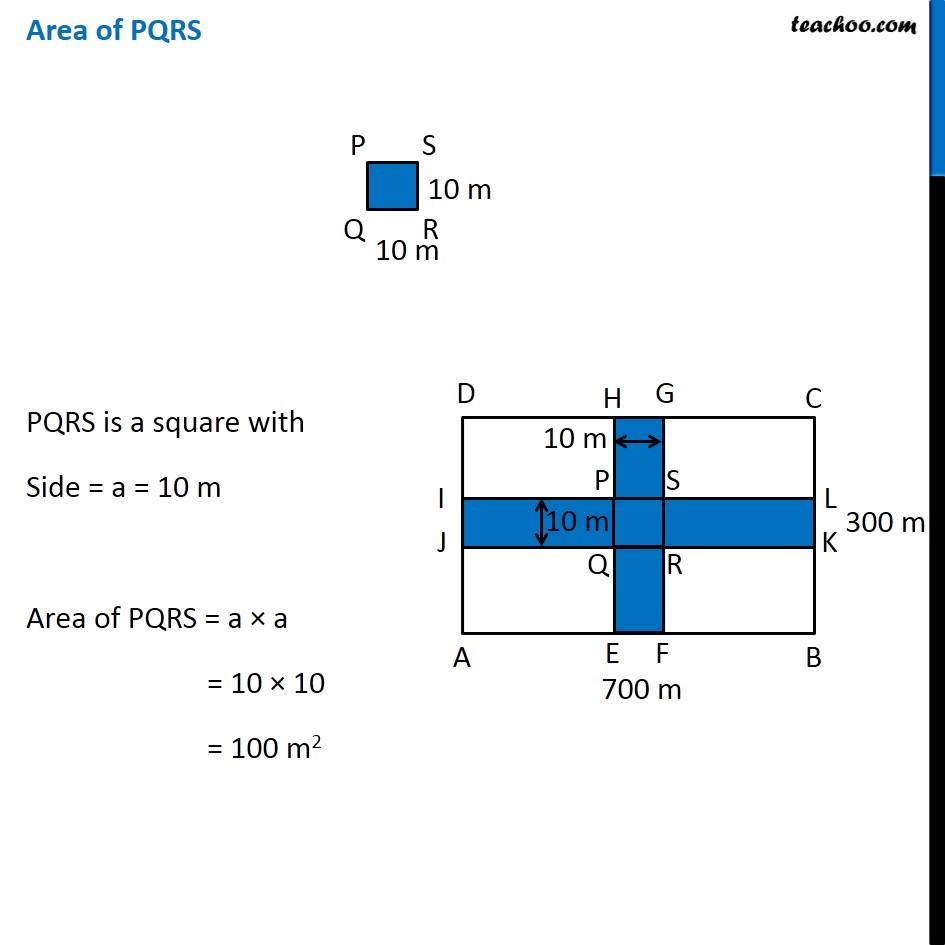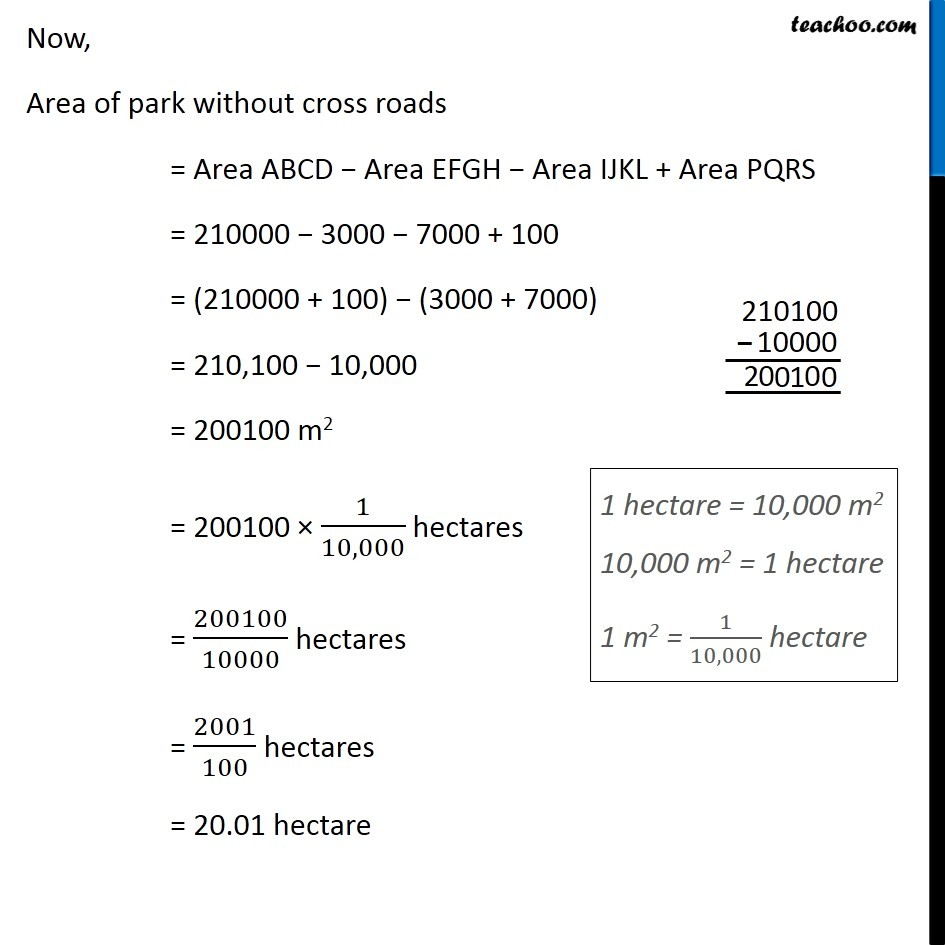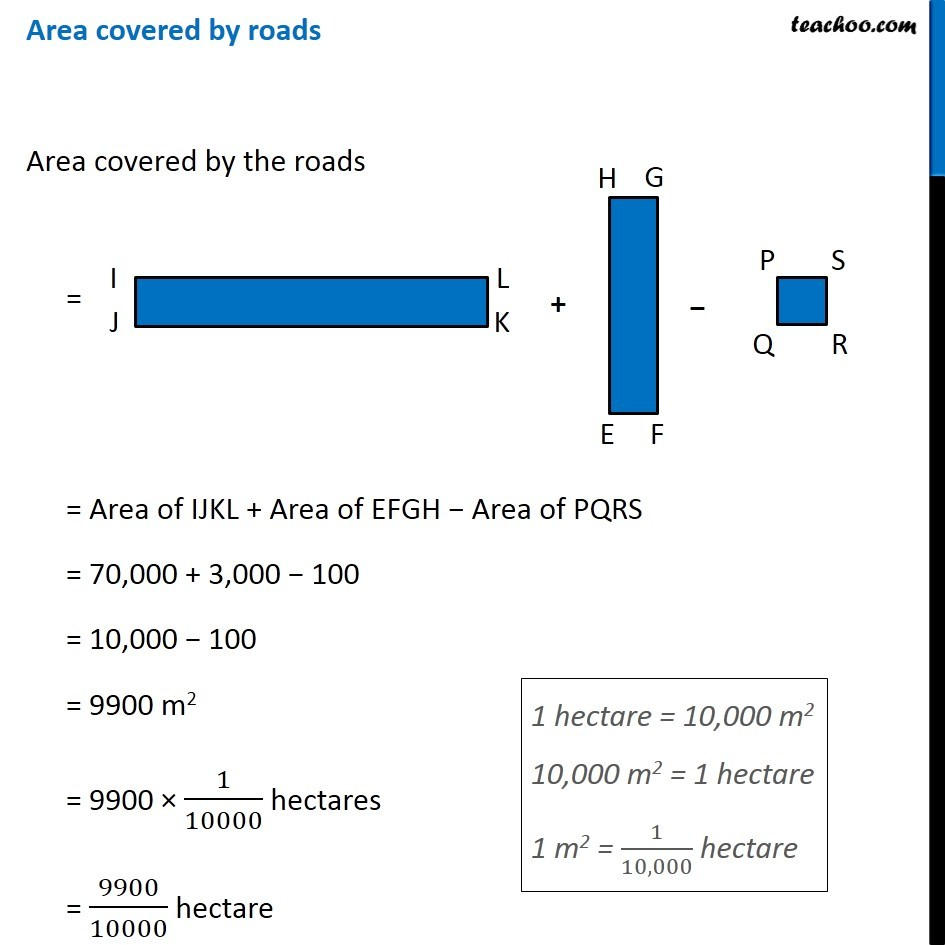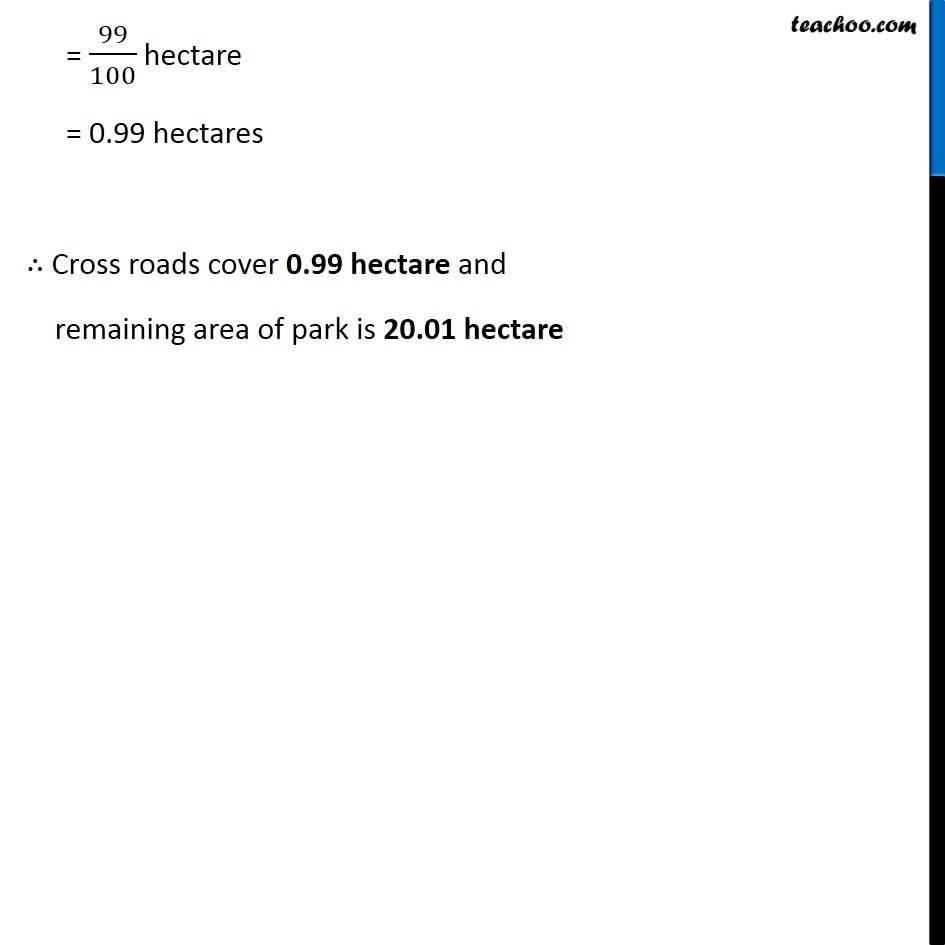1. Chapter 11 Class 7 Perimeter and Area
2. Concept wise
3. Finding Area of rectangle with cross roads

Transcript

Ex 11.4, 6 Two cross roads, each of width 10 m, cut at right angles through the centre of a rectangular park of length 700 m and breadth 300 m and parallel to its sides. Find the area of the roads. Also find the area of the park excluding cross roads. Give the answer in hectares. Our figure looks like We need to find area of park without the cross roads So, Area of park without cross roads = Area ABCD − Area EFGH − Area IJKL + Area PQRS Note: Here we add area PQRS because it gets subtracted twice Area of ABCD ABCD is a rectangle with Length = l = 700 m Breadth = b = 300 m Area of ABCD = l × b = 700 × 300 = 2,10,000 m2 Area of EFGH EFGH is a rectangle with Length = l = 10 m Breadth = b = 300 m Area of EFGH = l × b = 10 × 300 = 3,000 m2 Area of IJKL IJKL is a rectangle with Length = l = 700 m Breadth = b = 10 m Area of IJKL = l × b = 700 × 10 = 7,000 m2 Area of PQRS PQRS is a square with Side = a = 10 m Area of PQRS = a × a = 10 × 10 = 100 m2 Now, Area of park without cross roads = Area ABCD − Area EFGH − Area IJKL + Area PQRS = 210000 − 3000 − 7000 + 100 = (210000 + 100) − (3000 + 7000) = 210,100 − 10,000 = 200100 m2 = 200100 × 1/10,000 hectares = 200100/10000 hectares = 2001/100 hectares = 20.01 hectare Area covered by roads Area covered by the roads = Area of IJKL + Area of EFGH − Area of PQRS = 70,000 + 3,000 − 100 = 10,000 − 100 = 9900 m2 = 9900 × 1/10000 hectares = 9900/10000 hectare 1 hectare = 10,000 m2 10,000 m2 = 1 hectare 1 m2 = 1/10,000 hectare = 99/100 hectare = 0.99 hectares ∴ Cross roads cover 0.99 hectare and remaining area of park is 20.01 hectare

Finding Area of rectangle with cross roads

About the AuthorDavneet Singh
Davneet Singh is a graduate from Indian Institute of Technology, Kanpur. He has been teaching from the past 10 years. He provides courses for Maths and Science at Teachoo.